User’s Guide : Basic Data Analysis : Groups : Cross Correlations and Correlograms

Cross Correlations and Correlograms
This view displays the cross correlations of the first two series in the group. The cross correlations between the two series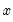and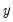are given by,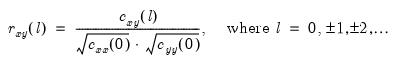(12.60)
and,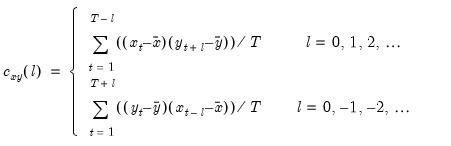(12.61)
As with the correlograms ( “Correlogram”), the computed cross correlation is an asymptotically consistent approximation as the means used in the computation are the overall means.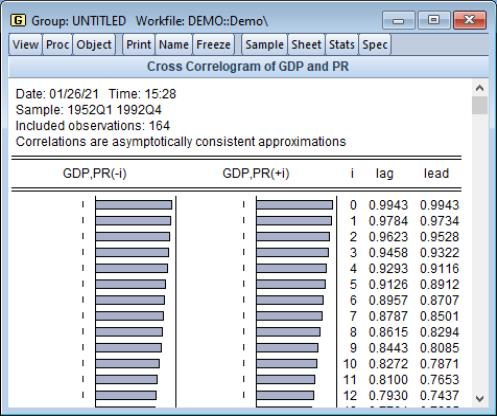Note that, unlike autocorrelations, cross correlations are not necessarily symmetric around lag 0.
The dotted lines in the cross correlograms are the approximate two standard error bounds computed as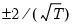.